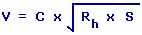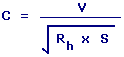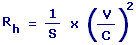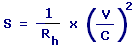Chezy Formula Calculators Enter value, select unit and click on calculate. Result will be displayed.

Calculate Mean Velocity
Calculate Chezy Coefficient
Calculate Slope

 Web www.calculatoredge.com

Calculate Mean VelocityV = Mean Velocity C = Chezy Coefficient Rh = Hydraulic Radius S = Slope
 Enter your values: Chezy Coefficient: Hydraulic Radius: Meter Centimeter Attometer Dekameter Decimeter Exameter Femtometer Foot Hectometer Inch Kilometer Megameter Micrometer Mile Millimeter Nanometer Petameter Picometer Yard Slope: Result: Mean Velocity: Meter/Second

Calculate Chezy CoefficientC = Chezy Coefficient V = Mean Velocity Rh = Hydraulic Radius S = Slope
 Enter your values: Mean Velocity: Meter / Second Foot / Second Centimeter / Hour Centimeter / Second Foot / Day Inch / Hour Inch / Second Kilometer / Second Knot Meter / Day Mile / Hour Mile / Second Millimeter / Second Yard / Second Hydraulic Radius: Meter Centimeter Attometer Dekameter Decimeter Exameter Femtometer Foot Hectometer Inch Kilometer Megameter Micrometer Mile Millimeter Nanometer Petameter Picometer Yard Slope: Result: Chezy Coefficient:Rh = Hydraulic Radius V = Mean Velocity C = Chezy Coefficient S = Slope
 Enter your values: Slope: Mean Velocity: Meter / Second Foot / Second Centimeter / Hour Centimeter / Second Foot / Day Inch / Hour Inch / Second Kilometer / Second Knot Meter / Day Mile / Hour Mile / Second Millimeter / Second Yard / Second Chezy Coefficient: Result: Hydraulic Radius: Meter

Calculate SlopeS = Slope V = Mean Velocity C = Chezy Coefficient Rh = Hydraulic Radius
 Enter your values: Hydraulic Radius: Meter Centimeter Attometer Dekameter Decimeter Exameter Femtometer Foot Hectometer Inch Kilometer Megameter Micrometer Mile Millimeter Nanometer Petameter Picometer Yard Mean Velocity: Meter / Second Foot / Second Centimeter / Hour Centimeter / Second Foot / Day Inch / Hour Inch / Second Kilometer / Second Knot Meter / Day Mile / Hour Mile / Second Millimeter / Second Yard / Second Chezy Coefficient: Result: Slope:LINKSDISCLAIMERCONTACT US